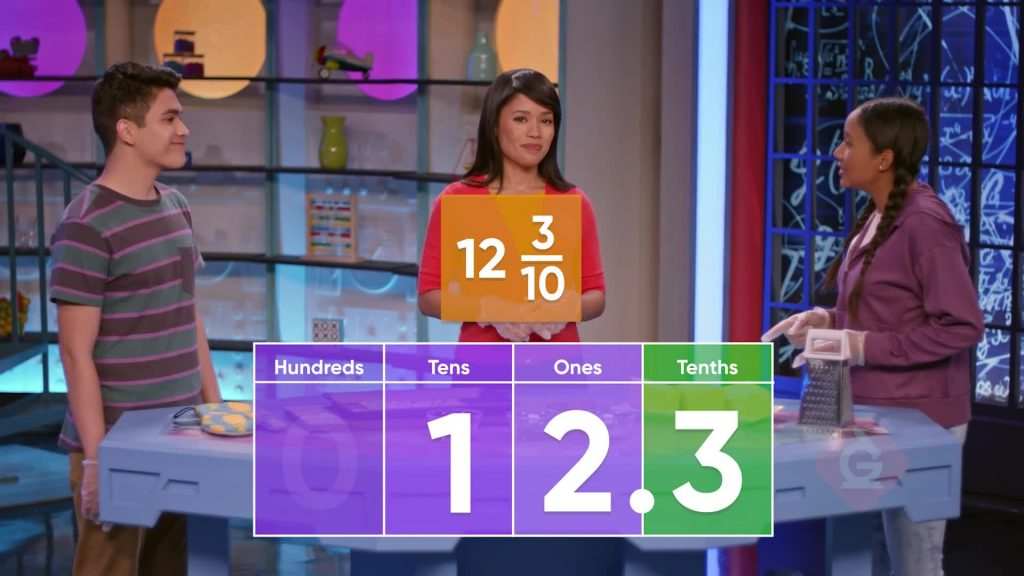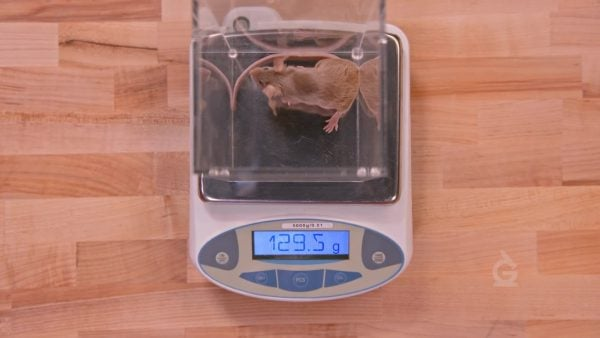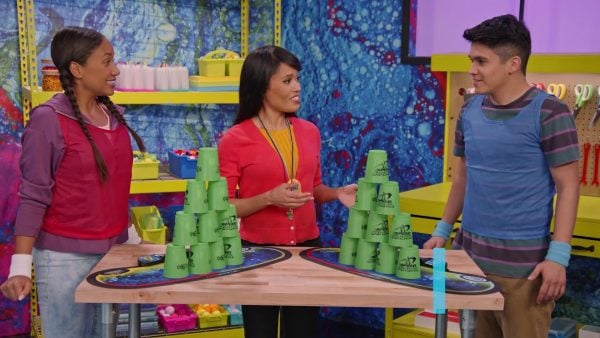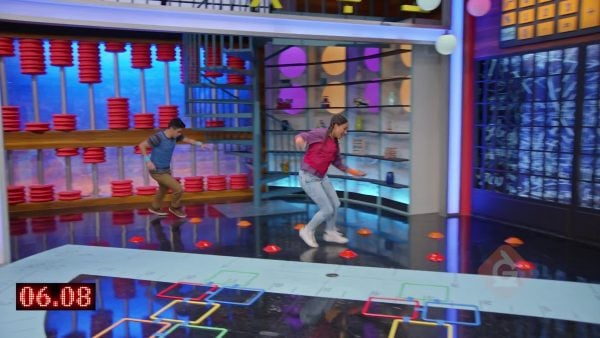Introduction to Decimals (Tenths & Hundredths) | 3rd, 4th, & 5th Grades
1%
It was processed successfully!WHAT IS INTRODUCTION TO DECIMALS (TENTHS & HUNDREDTHS)?

Decimal notation is an easy way to represent fractions with denominators of 10 or 100. Solving problems using decimal numbers can be much easier than solving those same problems using fractions.

To better understand decimals…

WHAT IS INTRODUCTION TO DECIMALS (TENTHS & HUNDREDTHS)?. Decimal notation is an easy way to represent fractions with denominators of 10 or 100. Solving problems using decimal numbers can be much easier than solving those same problems using fractions. To better understand decimals…

## LET’S BREAK IT DOWN!Let’s say you want to make cheesy sticks for a party and you need 12 [ggfrac]3/10[/ggfrac] loaves of bread. You can represent that number with a decimal. You know how to write 12 with a 1 in the tens place and a 2 in the ones place. You can put a decimal point after the 2 in the ones place. The next place to the right is the tenths place. You can write 3 in the tenths place to represent 0.3. 12.3 is 12 310 written as a decimal. Try this one yourself. To double the cheesy stick recipe, you need 24 [ggfrac]6/10[/ggfrac] loaves of bread. How can you represent that number with a decimal?

Cheesy Bread Let’s say you want to make cheesy sticks for a party and you need 12 [ggfrac]3/10[/ggfrac] loaves of bread. You can represent that number with a decimal. You know how to write 12 with a 1 in the tens place and a 2 in the ones place. You can put a decimal point after the 2 in the ones place. The next place to the right is the tenths place. You can write 3 in the tenths place to represent 0.3. 12.3 is 12 310 written as a decimal. Try this one yourself. To double the cheesy stick recipe, you need 24 [ggfrac]6/10[/ggfrac] loaves of bread. How can you represent that number with a decimal?

### Pet MiceLet’s say you want to compare the weights of your two pet mice. One mouse weighs 129.3 grams. The second mouse weighs 129.5 grams. You can use place value to compare those two numbers. Start at the greatest place to compare. Both numbers have 1 in the hundreds place, 2 in the tens place, and 9 in the ones place. To find which number is greater, compare the digits in the tenths place. 5 tenths is greater than 3 tenths, so 129.5 grams is greater than 129.3 grams. Try this one yourself. One of April's pet mice weighs 127.4 grams. The other mouse weighs 127.1 grams. Which mouse is the heaviest?

Pet Mice Let’s say you want to compare the weights of your two pet mice. One mouse weighs 129.3 grams. The second mouse weighs 129.5 grams. You can use place value to compare those two numbers. Start at the greatest place to compare. Both numbers have 1 in the hundreds place, 2 in the tens place, and 9 in the ones place. To find which number is greater, compare the digits in the tenths place. 5 tenths is greater than 3 tenths, so 129.5 grams is greater than 129.3 grams. Try this one yourself. One of April's pet mice weighs 127.4 grams. The other mouse weighs 127.1 grams. Which mouse is the heaviest?

### Cup-StackingLet’s say you and your friend enter a cup-stacking contest. It takes you 9.04 seconds to stack the cups. It takes your friend 9.26 seconds to stack the cups. Who won the contest? First we compare the ones. 9 = 9, so we compare the tenths: 0 < 2. So, 9.04 < 9.26. You took less time, so you won the contest. Try this one yourself. It took April 10.53 seconds to stack the cups. It took Marcos 10.06 seconds to stack the cups. Who took the least amount of time to stack the cups?

Cup-Stacking Let’s say you and your friend enter a cup-stacking contest. It takes you 9.04 seconds to stack the cups. It takes your friend 9.26 seconds to stack the cups. Who won the contest? First we compare the ones. 9 = 9, so we compare the tenths: 0 < 2. So, 9.04 < 9.26. You took less time, so you won the contest. Try this one yourself. It took April 10.53 seconds to stack the cups. It took Marcos 10.06 seconds to stack the cups. Who took the least amount of time to stack the cups?

### Obstacle CourseLet’s say Jill and Niko run an obstacle course. The record at the course is 16.3 seconds. Jill ran the course in 16.37 seconds. 16.3 has 0 hundredths. 16.37 has 7 hundredths. So, 16.37 > 16.3. Jill did not break the record. Niko runs the course in 16.29 seconds. 16.29 has 2 tenths, which is less than 3 tenths. 16.29 < 16.3. Niko broke the record. Try this one yourself. Another course has a record time of 14.02 seconds. You ran that course in 14.2 seconds. Did you break the record?

Obstacle Course Let’s say Jill and Niko run an obstacle course. The record at the course is 16.3 seconds. Jill ran the course in 16.37 seconds. 16.3 has 0 hundredths. 16.37 has 7 hundredths. So, 16.37 > 16.3. Jill did not break the record. Niko runs the course in 16.29 seconds. 16.29 has 2 tenths, which is less than 3 tenths. 16.29 < 16.3. Niko broke the record. Try this one yourself. Another course has a record time of 14.02 seconds. You ran that course in 14.2 seconds. Did you break the record?

## INTRODUCTION TO DECIMALS (TENTHS & HUNDREDTHS) VOCABULARY

Decimal
A number whose whole number part is separated from the fractional part by a decimal point. The digits following the decimal point show a value that is less than 1.
Place value
The value of a digit based on its position in a number.
Digit
A symbol used to make a numeral. 0, 1, 2, 3, 4, 5, 6, 7, 8, 9 are the 10 digits we use in our number system.
Tenth
The tenths place shows the number of equal pieces out of 10.
Decimal point
A dot that is used to separate the ones place from the tenths place.
Less than
Shows that one value is less than another. The less than symbol (<) is used to show this relationship.
Shows that one value is greater than another. The greater than symbol (>) is used to show this relationship.
The hundredths place shows the number of equal pieces out of 100.

## INTRODUCTION TO DECIMALS (TENTHS & HUNDREDTHS) DISCUSSION QUESTIONS

### Draw a picture to represent 0.6.

A shape divided into 10 equal parts with 6 parts shaded.

### How do you compare 0.2 and 0.4?

Both the digit 2 and the digit 4 are in the tenths place. 4 is greater than 2, so 4 tenths is greater than 2 tenths. 0.4 > 0.2

### Draw a picture to represent 0.07.

A shape divided into 100 equal parts with 7 parts shaded.

### How do you compare 0.78 and 0.73?

First, I compare the digit in the tenths place. Both numbers have the digit 7 in the tenths place. So I compare the digits in the hundredths place. 0.78 has the digit 8 in the hundredths place. 0.73 has the digit 3 in the hundredths place. 8 hundredths is greater than 3 hundredths. 0.78 is greater than 0.73.

### Jason says that 0.09 > 0.3 because 9 is greater than 3. Is he correct? Explain.

Jason is not correct. 0.3 is equal to 0.30. Now compare 0.09 and 0.30. 30 hundredths is greater than 9 hundredths, so 0.30 is greater than 0.09. 0.3 > 0.09
X

## Success

We’ve sent you an email with instructions how to reset your password.
Ok